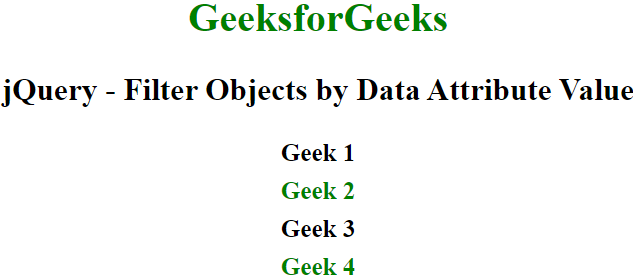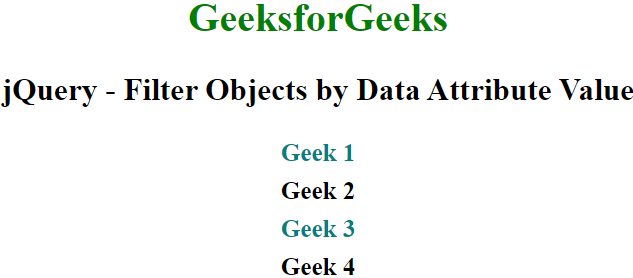# How to Filter Objects by Data Attribute Value in jQuery ?

• Last Updated : 02 Mar, 2022

In this article, we will learn how to filter objects by their data attribute value in jQuery. There are two approaches that can be taken to achieve this:

Approach 1:  Using the this property and filter(), data() and css() methods in the jQuery library. There are four division elements that are defined in the following example, each having some data attribute with its corresponding value. We apply the filter() method to each division element which takes an anonymous function as its parameter. This anonymous function returns either the boolean true or false, depending on whether the value of the data attribute of each division element is equal to a particular string ( string is “valid”). To check this, the data() method is used which takes the attribute name after the data- prefix as a string parameter.

After this, we chain the css() method with the filter() method to apply some styling on all the filtered elements or objects based on the functionality discussed above.

Example: In the following example, the data-attribute has been named as data-geek and all the filtered elements have been styled with a font color of green.

## HTML

 ```<``html``>`` ` `<``head``>``    ``<``script` `src``=``"https://ajax.googleapis.com/ajax/libs/jquery/3.5.1/jquery.min.js"``>``    ```` ` `    ````    ``<``style``>``        ``body {``            ``text-align: center;``        ``}`` ` `        ``p {``            ``font-size: 25px;``            ``font-weight: bold;``        ``}`` ` `        ``div {``            ``font-size: 20px;``            ``font-weight: bold;``        ``}`` ` `        ``div:not(:last-child) {``            ``margin-bottom: 0.5rem;``        ``}``    `````` ` `<``body``>``    ``<``h1` `style``=``"color:green"``>GeeksforGeeks`` ` `    ``<``p``>jQuery - Filter Objects by Data Attribute Value`` ` `    ``<``div` `class``=``"geek-division"` `data-geek``=``"invalid"``>``        ``Geek 1``    ````    ``<``div` `class``=``"geek-division"` `data-geek``=``"valid"``>``        ``Geek 2``    ````    ``<``div` `class``=``"geek-division"` `data-geek``=``"invalid"``>``        ``Geek 3``    ````    ``<``div` `class``=``"geek-division"` `data-geek``=``"valid"``>``        ``Geek 4``    ```` ` `    ``<``script` `type``=``"text/javascript"``>`` ` `        ``// Select all div with the class``        ``// attribute "division"``        ``\$('div[class*="division"]')``            ``.filter(function () {``                ``return \$(this).data("geek") == "valid";``            ``}).css("color", "green");``    `````` ` `  `

Output:Approach 2: Using the filter() and css() methods in the jQuery library. This approach is quite similar to the previous approach but the syntax is slightly cleaner and condensed. We are checking if the value of the data attribute of each division element is equal to the string “open” instead of the string “valid” to filter the division elements.

Example: In the following example, the data-attribute has been named as data-state and all the filtered elements have been styled with a font colour of teal.

## HTML

 ```<``html``>`` ` `<``head``>``    ``<``script` `src``=``"https://ajax.googleapis.com/ajax/libs/jquery/3.5.1/jquery.min.js"``>``    ```` ` `    ````    ``<``style``>``        ``body {``            ``text-align: center;``        ``}`` ` `        ``p {``            ``font-size: 25px;``            ``font-weight: bold;``        ``}`` ` `        ``div {``            ``font-size: 20px;``            ``font-weight: bold;``        ``}`` ` `        ``div:not(:last-child) {``            ``margin-bottom: 0.5rem;``        ``}``    `````` ` `<``body``>``    ``<``h1` `style``=``"color: green"``>GeeksforGeeks`` ` `    ``<``p``>jQuery - Filter Objects by Data Attribute Value`` ` `    ``<``div` `class``=``"geek-division"` `data-state``=``"open"``>``        ``Geek 1``    ````    ``<``div` `class``=``"geek-division"` `data-state``=``"closed"``>``        ``Geek 2``    ````    ``<``div` `class``=``"geek-division"` `data-state``=``"open"``>``        ``Geek 3``    ````    ``<``div` `class``=``"geek-division"` `data-state``=``"closed"``>``        ``Geek 4``    ```` ` `    ``<``script` `type``=``"text/javascript"``>``         ` `        ``// Select all div elements with the ``        ``// class attribute containing "division"``        ``\$("div[class*=division]")``            ``.filter("div[data-state=open]")``            ``.css("color", "teal");``    `````` ` ``

Output:My Personal Notes arrow_drop_up